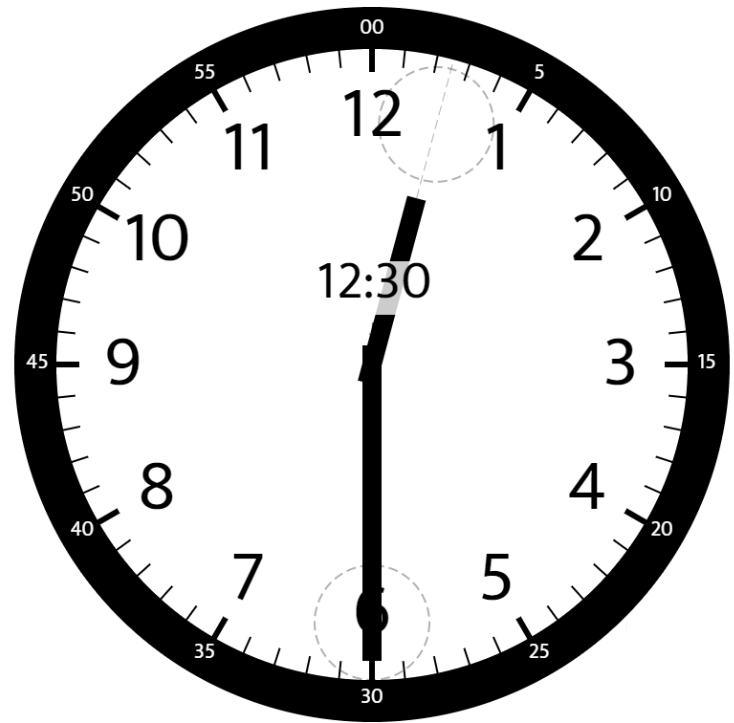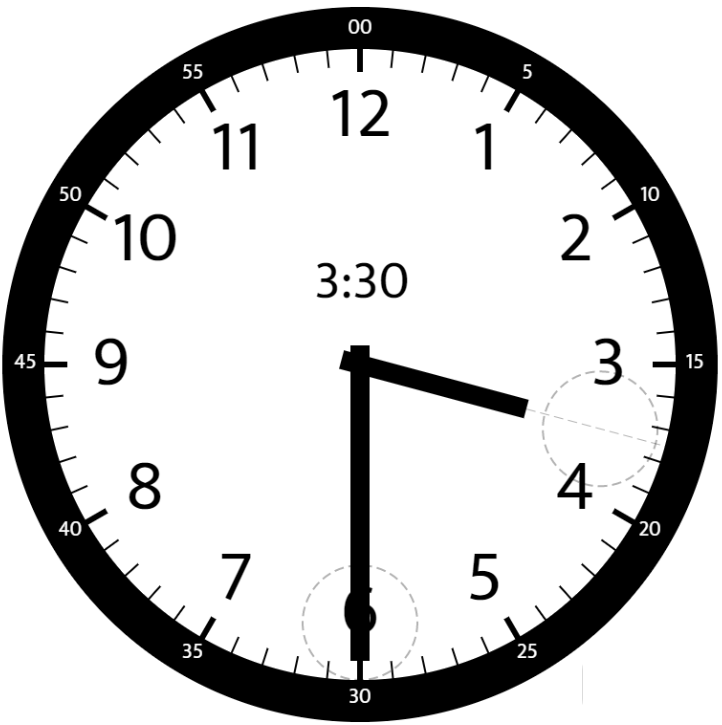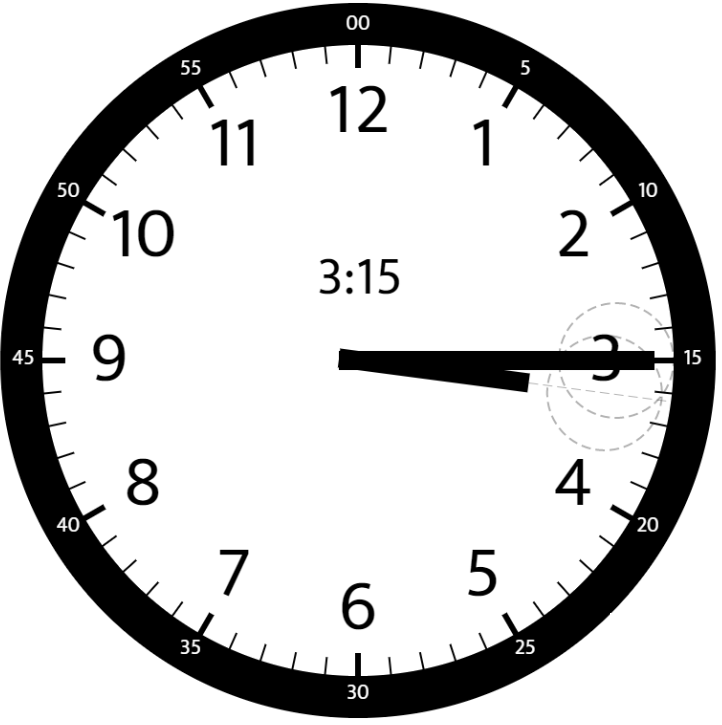# GeetCode Hub

Given two numbers, `hour` and `minutes`. Return the smaller angle (in degrees) formed between the `hour` and the `minute` hand.

Example 1:```Input: hour = 12, minutes = 30
Output: 165
```

Example 2:```Input: hour = 3, minutes = 30
Output: 75
```

Example 3:```Input: hour = 3, minutes = 15
Output: 7.5
```

Example 4:

```Input: hour = 4, minutes = 50
Output: 155
```

Example 5:

```Input: hour = 12, minutes = 0
Output: 0
```

Constraints:

• `1 <= hour <= 12`
• `0 <= minutes <= 59`
• Answers within `10^-5` of the actual value will be accepted as correct.

class Solution { public double angleClock(int hour, int minutes) { } }